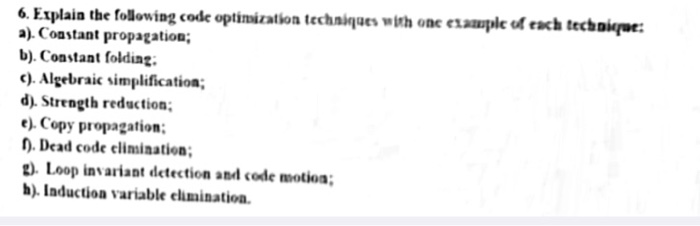# Answered! Explain the following code optimization techniques with one example of each a) Constant propagation: b) Constant…Explain the following code optimization techniques with one example of each a) Constant propagation: b) Constant folding: c) Algebraic simplification: d) Strength reduction: e) Copy propagation: f) Dead code elimination: g) Loop invariant election and code motion: h) Induction variable elimination.

a> Constant Propagation:

If The value of a variable is a constant ,Then replace the variable by the constant.

Example:

Don't use plagiarized sources. Get Your Custom Essay on
Answered! Explain the following code optimization techniques with one example of each a) Constant propagation: b) Constant…
GET AN ESSAY WRITTEN FOR YOU FROM AS LOW AS \$13/PAGE

In the code below, the value of x can be propagated to the use of x.

```x = 3; here we initialize variable x with value 3,Now it means x is 3
y = x + 4;
```

b> Constant folding:

Expression with constant operands can be evaluated at compile time rather than computing them at runtime.

Example:

```int Multiplication ()// The expression (3 * 2) can be evaluated at compile time and replaced with the constant 6.
{
return 3 * 2;
}

```

Below is the code fragment after constant folding.

```int Multiplication()
```
```{
return 6;
}

```

c> Algebraic simplification:

Algebraic simplification use algebraic properties of operators or particular operator – operand combinations to simplify expressions.

Example:

1. a+0 = 0+a =a-0 = a // Here we add zero to a or add a to zero or substract 0 from a result will be a.

2. a*1 = 1*a = a/1 = a // Here we multiply a with 1 or multiply 1 with a or divide a by 1 result will be a.

above example show algebraic simplification write a instead of a-0 or a*1 .

d> Strength reduction :

In this techniques expensive operations are replaced with equivalent but less expensive operations.

Example:

i*2 = 2*i = i+i

i/2 = (int)( i* 0.5) //here i is integer so 1/2 is written as 0.5 and whole is cast as int.

e> Copy propagation:

It is the process of replacing the occurrences of targets of direct assignments with their values.

Example:

Below code:

a =b

c= 6+a

can be written as , c= 6+b , because b is assign to a.

It is techniques to remove code that does not affect the results

Example:

``` int main() {
int a = 5;
int b = 6;
int c;
c = a * b;
if (0) {   // Here is if(0) means it is always false so inside if block code does not execute so we can remove code inside if block.This technique is dead code elimination.
cout<<"Value of c is"<<c;
}
return c;
}
```

g> Loop invariant detection and code motion

If the result of a statement or expression does not change within a loop, and it has no external side-effect

Computation can be moved to outside of the loop.

If we consider the following code sample, two optimizations can be easily applied.

```for (int i = 0; i < n; i++) {
x = y + z;
a[i] = 6 * i + x * x;
}
```

The calculation x = y + z and x * x can be moved outside the loop since within they are loop-invariant code, so the optimized code will be something like this:

```x = y + z;
t1 = x * x;
for (int i = 0; i < n; i++) {
a[i] = 6 * i + t1;
}
```

h> Induction variable elimination:

Some loops contain two or more induction variable that can be combined into one induction variable .

Example:

Below program has three induction variables (i1, i2, and i3) that can be replaced with one induction variable, thus eliminating two induction variables.

```int a;
int b;

void assign ()
{
int i1, i2, i3;

for (i1 = 0, i2 = 0, i3 = 0; i1 < 10; i1++)
a[i2++] = b[i3++];
return;
}
```

The code fragment below shows the loop after induction variable elimination.

```int a;
int b;

void assign()
{
int i1;

for (i1 = 0; i1 < 10; i1++)
a[i1] = b[i1];
return;
}```